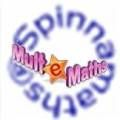# The art of Math

Over time there has always been one defining factor at schools: most kids have ‘reserved’ feelings towards math. Maybe it’s the number factor, maybe it’s the equations or maybe it’s the fact that x + y shouldn’t equal anything because they are letters.

But Manzana is proud to offer the hard working math teachers of this country two very interactive and clever solutions looking to make maths easier.The Mult-e-Maths Toolbox consists of a workspace and tools that you can combine and use to create learning experiences customised for your class.

Designed for use on the interactive whiteboard, the Toolbox will enhance maths teaching and help you to demonstrate maths concepts that cannot easily be shown in any other way.

The specialised maths tools are organised into eight mathematical strands: numbers, calculations, fractions, measures, shape and space, handling data, probability and solving problems. All the tools are easily accessible at any point whilst using the program.

The Toolbox is a versatile resource where you can plan, create and save your own lessons. Each Toolbox document contains six blank sheets that you can use to organise lessons. You can even email your saved lessons to colleagues. The Toolbox can be used independently or alongside a range of Mult-e-Maths titles to develop extension or support activities.

Benefits:
• Differentiated whole-class material will engage all children
• The Toolbox reinforces student’s understanding of key mathematical concepts
• Promotes a greater understanding of mathematical concepts through the use of highly engaging and effective visual tools

Features
• 8 Toolboxes with over 60 interactive tools in total
• All tools are available to hand on one screen for easy access
• Provides a wealth of ideas for starter and lesson activities
• Ability to plan your activities at home and email them to yourself at school.

Spinnamaths is an advanced and highly detailed mathematics learning solution. Using Spinnamaths, teachers can save time and effort explaining complex concepts, by using powerful illustrative and experiential techniques.
Spinnamaths is a family of interactive spreadsheet-based concept exemplars. These exemplars allow teachers and students to experiment with variables, and observe the consequences.

Spinnamath’s 60 interactive exemplars include the following key math concepts:

• The effect of varying coefficients on functions
• The Newton-Raphson method of finding roots
• The connection between tangents and the derivative of a function
• The side by side comparison of a function and its derivative
• The conjugate nature of complex roots of certain polynomial equations
• The approximation of areas under curves using Simpson’s rule
• The concept of a volume of solid of revolution
• The concept of an inverse
• The concept of optimisation
• The concept of area approximation using rectangles
• The concept of areas under curves
• The first principles understanding of the derivative
• The construction of the least squares line of best fit
• Superposition
• Polar and conic representations
• Mortgages and annuities
• Projectile and simple harmonic motion
• The special nature of e
• The concept of a complex number in Cartesian and polar form
• The roots of unity and Julia tours
• The hypothesis testing on sample means
• Investigations into the sine and cosine rule and bearings
• Basic understandings in coordinate geometry
• Special investigations such as the Euler line and Lewis Carroll’s day-finder
• Basic understandings of logarithms and percentages

There are many more. The key feature of all the sheets is the concept and not the graph.
Spinnamaths has an additional feature which is to continually beg the ‘what if’ question. If I push this coefficient, what happens to the graph? If I start with this estimate of a root what happens to successive Newton-Raphson tangents? What is the effect of a high interest rate on a mortgage? Why does Simpson’s rule give exact answers for a polynomial of degree 3?

Although both solutions differ in content, their abilities to take the subject of maths and create a format for understanding it is where they come together. Both solutions provide ample examples and template for use when trying to explain those difficult problems to your students- they also add interactivity to your lesson, keeping your students engaged for longer.

For further information concerning Spinnamaths or Mult-e-Maths email Manzana or give us a call on 0800 200 121.